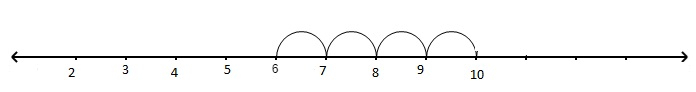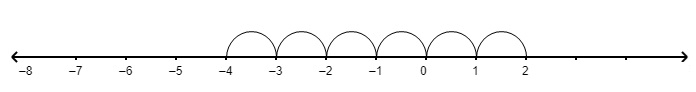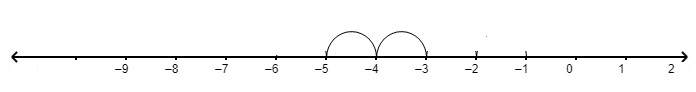# Using the number line, write the integer which is(i) 4 more than 6(ii) 5 more than $-6$(iii) 6 less than 2(iv) 2 less than $-3$

Given :

The given terms are

(i) 4 more than 6
(ii) 5 more than $-6$
(iii) 6 less than 2
(iv) 2 less than $-3$

To do :

We have to write the numbers using the number line.

Solution :

(a) 4 more than 6

Step 1: First mark 6.

Step 2: Move 4 steps to the right.So, 4 more than 6 is 10.

(b) 5 more than $-6$

Step 1: First mark $-6$.

Step 2: Move 5 steps to the right.So, 5 more than $-6$ is $-1$.

(c) 6 less than 2

Step 1: First mark 2.

Step 2: Move 6 steps to the left.So, 6 less than 2 is $-4$.

(d) 2 less than $-3$

Step 1: First mark $-3$.

Step 2: Move 2 steps to the left.So, 2 less than $-3$ is $-5$.